PSA9CC5 - Student-Facing Tasks---7.1 Lesson 4: Scaled Relationships: Student Facing Tasks (7.G.A.1)
Part A)

Each of these polygons is a scaled copy of the others.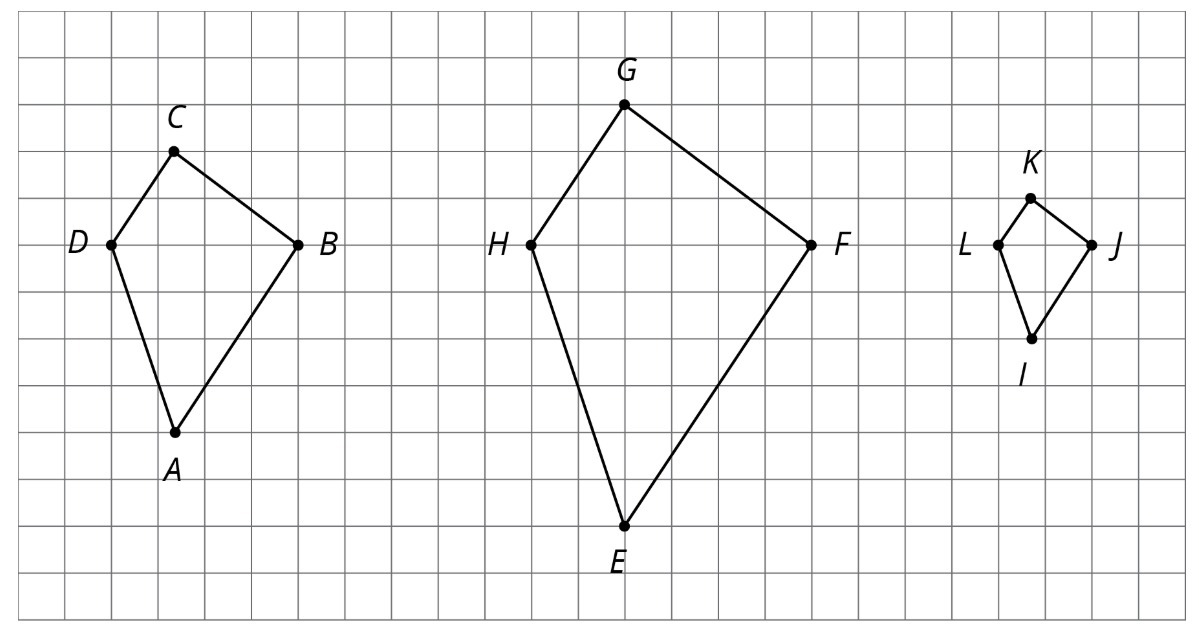Name two pairs of corresponding angles. What can you say about the sizes of these angles?

Part B)

Check your prediction by measuring at least one pair of corresponding angles using a protractor. Record your measurements to the nearest 5.

Part A)

Each of these polygons is a scaled copy of the others. You already checked their corresponding angles.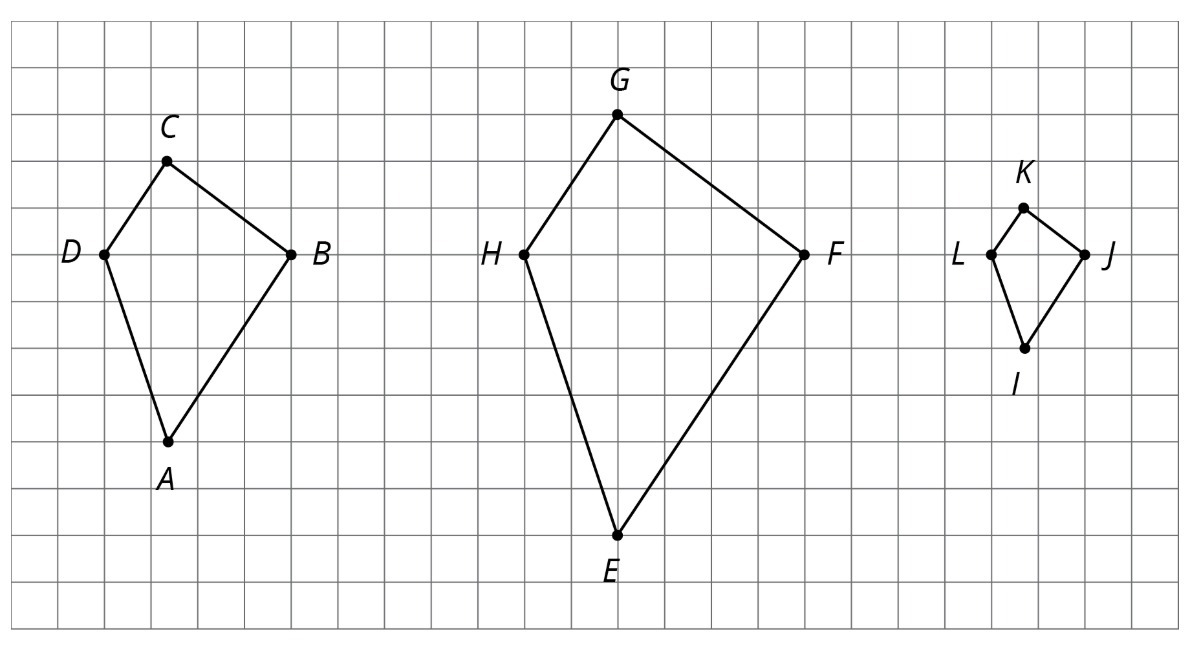The side lengths of the polygons are hard to tell from the grid, but there are other corresponding distances that are easier to compare. Identify the distances in the other two polygons that correspond to DB and AC, and record them in the table.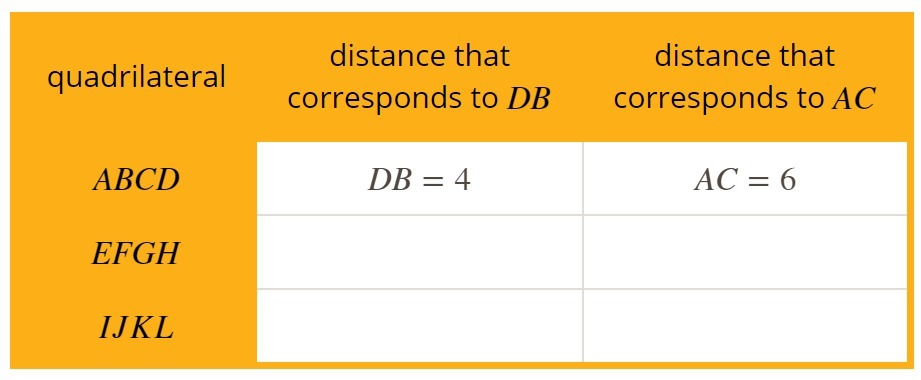Create and fill in the table using the table button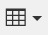Part B)

Look at the values in the table. What do you notice?

Part C)

The larger figure is a scaled copy of the smaller figure.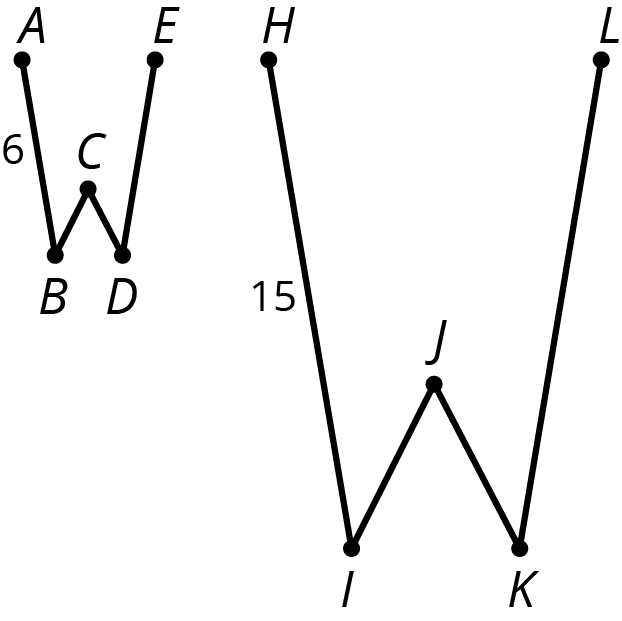If AE=4, how long is the corresponding distance in the second figure?

Type your answer below as a number (example: 5, 3.1, 4 1/2, or 3/2):
Part D)

Part E)

If IK=5, how long is the corresponding distance in the first figure?

Type your answer below as a number (example: 5, 3.1, 4 1/2, or 3/2):
Part F)

Part A)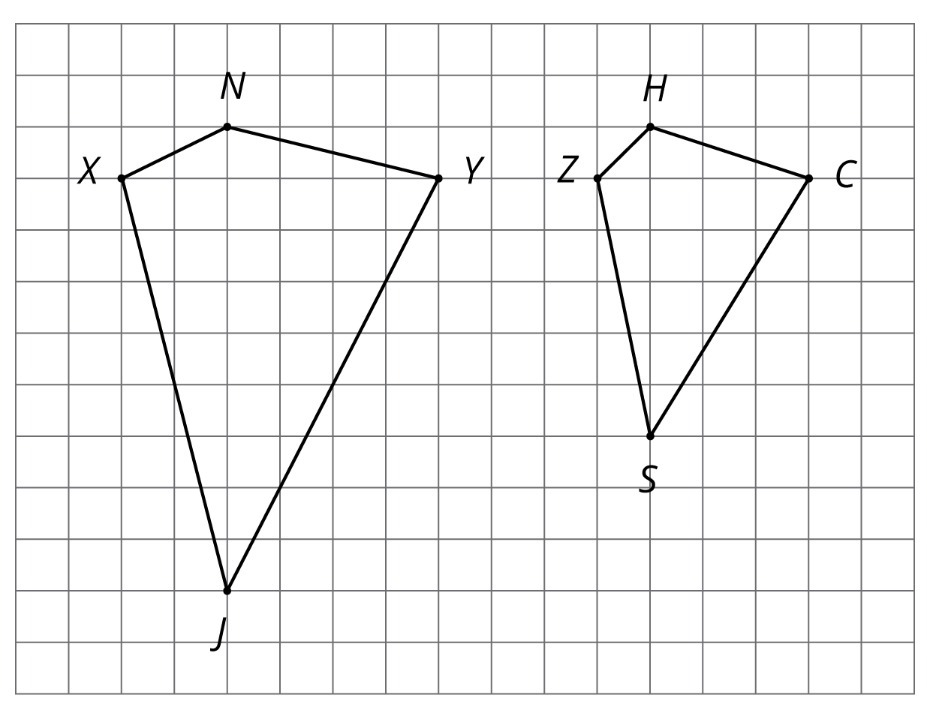Mai says that Polygon ZSCH is a scaled copy of Polygon XJYN, but Noah disagrees. Do you agree with either of them?

Select one:
Part B)

Part C)

Record the corresponding distances in the table.What is the length of XY?

Type your answer below as a number (example: 5, 3.1, 4 1/2, or 3/2):
Part D)

What is the length of JN?

Type your answer below as a number (example: 5, 3.1, 4 1/2, or 3/2):
Part E)

What is the length of ZC?

Type your answer below as a number (example: 5, 3.1, 4 1/2, or 3/2):
Part F)

What is the length of SH?

Type your answer below as a number (example: 5, 3.1, 4 1/2, or 3/2):
Part G)

Measure at least three pairs of corresponding angles in XJYN and ZSCH using a protractor. Record your measurements to the
nearest 5. What do you notice?

Part H)

Part I)Kiran says that Polygon EFGH is a scaled copy of ABCD, but Lin disagrees. Do you agree with either of them?

Select one:
Part J)

Here are two pictures of a bird. Find evidence that one picture is not a scaled copy of the other. Be prepared to explain your reasoning.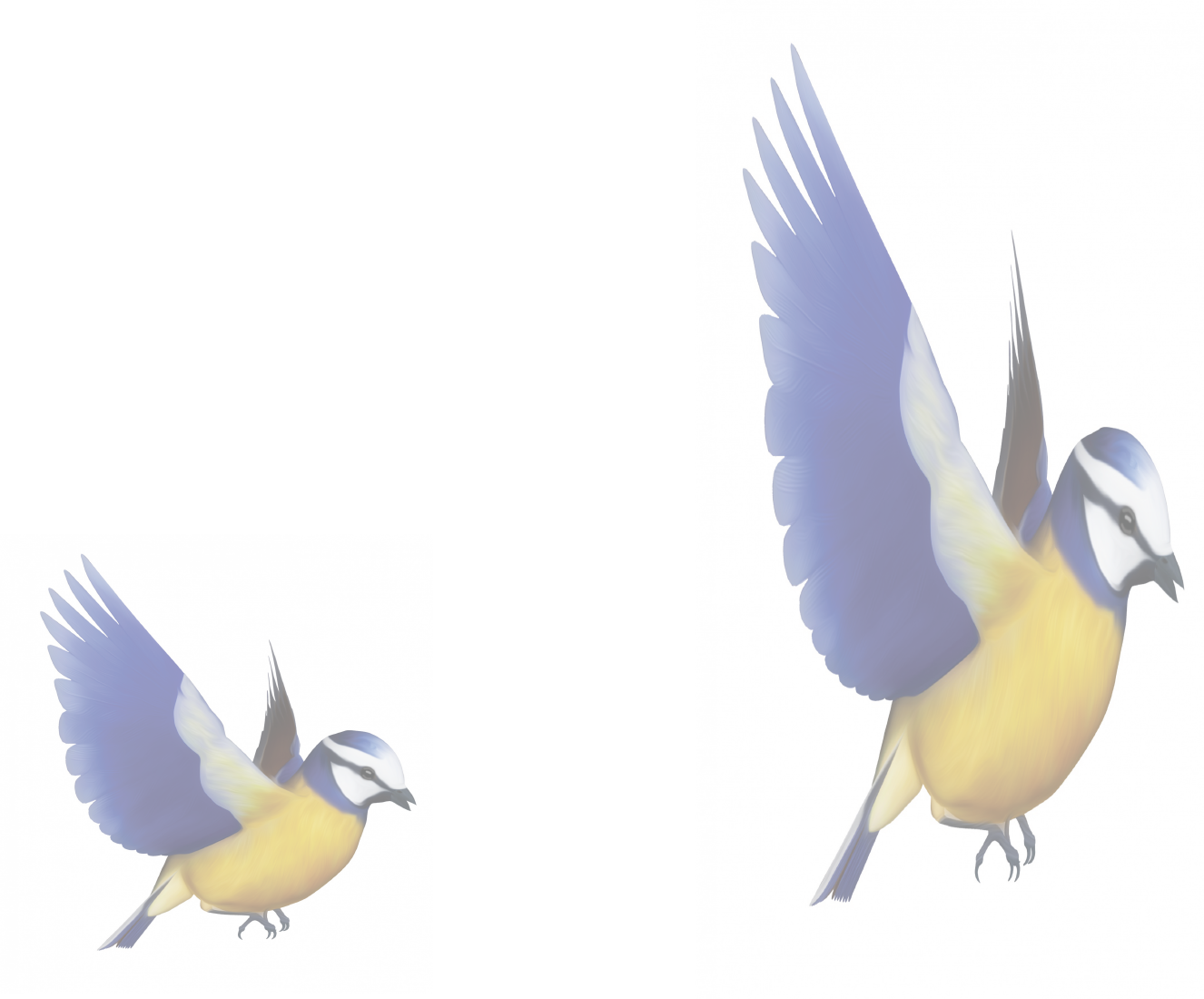Part A)

Here are two polygons on a grid.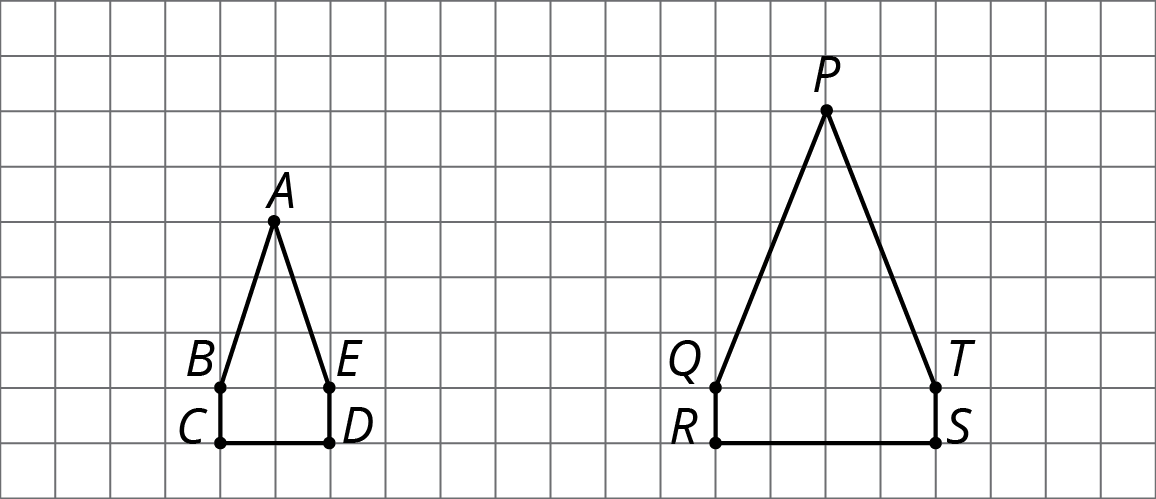Is PQRST a scaled copy of ABCDE?